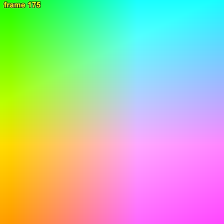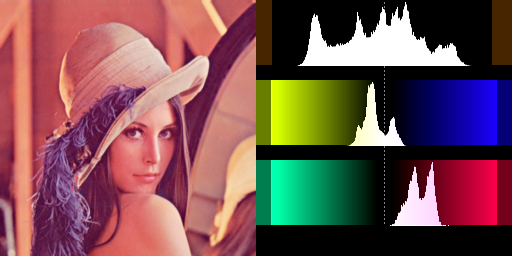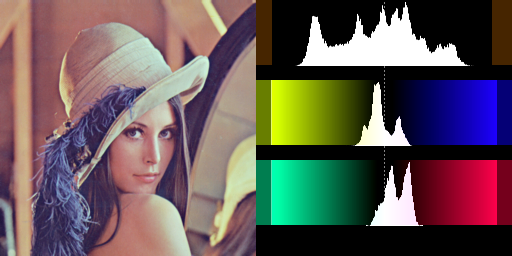# ColorYUV

ColorYUV has many different methods of changing the color and luminance of your images. Gain, offset, gamma and contrast can be set independently on each channel. Also included are two 'auto' modes, an analysis function and a color gamut generator.

All settings for this filter are optional. All values are defaulting to "0" or false.

RGBAdjust works in a similar manner for RGB clips.

## Syntax and Parameters

ColorYUV(clip [,
float gain_y, float off_y, float gamma_y, float cont_y,
float gain_u, float off_u, float gamma_u, float cont_u,
float gain_v, float off_v, float gamma_v, float cont_v,
string levels, string opt, bool showyuv, bool analyze,
bool autowhite, bool autogain, bool conditional ] )

ColorYUV(clip [,
float gain_y, float off_y, float gamma_y, float cont_y,
float gain_u, float off_u, float gamma_u, float cont_u,
float gain_v, float off_v, float gamma_v, float cont_v,
string levels, string opt, bool showyuv, bool analyze,
bool autowhite, bool autogain, bool conditional,
int bits, bool showyuv_fullrange, bool f2c, string condvarsuffix ] )
AVS+

float  gain_y = 0
float  gain_u = 0
float  gain_v = 0
A multiplier for the Y, U or V value.
If gain_y = k, then Y becomes Y · (k / 256 + 1) – for example:
(TL;DR see Parameter Rescaling below)
gain_x multiplier
-256 (-256 / 256) + 1 0.0 (no output)
-128 (-128 / 256) + 1 0.5 (darker)
0 (0 / 256) + 1 1.0 (no change)
256 (256 / 256) + 1 2.0 (brighter)
512 (512 / 256) + 1 3.0
• gain_x is valid down to -256, where a gain of 0.0 is applied.
• Although it is possible, it doesn't make sense to apply this setting to the chroma of the signal.
• In order to confuse you, in the filter Tweak this setting is called contrast.
float  off_y = 0
float  off_u = 0
float  off_v = 0
Adds an offset to the luma or chroma values.
• An off_x of 16 will add 16d to all pixel values.
• An off_x of -32 will subtract 32d from all pixel values.
float  gamma_y = 0
float  gamma_u = 0
float  gamma_v = 0
Adjusts gamma of the specified channel,
as X(1/g) where g=(gamma_x + 256) / 256 – for example:
(TL;DR see Parameter Rescaling below)
gamma_x gamma
-256 (-256 / 256) + 1 0.01
-128 (-128 / 256) + 1 0.5 (darker)
0 (0 / 256) + 1 1.0 (no change)
256 (256 / 256) + 1 2.0 (lighter)
512 (512 / 256) + 1 3.0
• gamma_x is valid down to -256, where a minimal gamma of 0.01 is applied (gamma cannot be zero)
• Note: gamma for chroma is not implemented (gamma_u and gamma_v are dummy parameters).
• AVS+ will operate on TV range instead of the default PC range when the input clip is known to be in TV range:
• levels="TV->PC", or levels="TV",
• or opt="coring" and levels is not "PC->TV" or "PC->TV.Y"
float  cont_y = 0
float  cont_u = 0
float  cont_v = 0
Like gain_X, a multiplier for the value, but stretches or shrinks the signal from the center (128d).
This is most useful for the chroma components, where it translates to saturation.
If cont_y = k, then Y becomes (Y-128) · k / 256 + 128 – for example:
(TL;DR see Parameter Rescaling below)
cont_x contrast
-256 (-256 / 256) + 1 0.0 (zero contrast)
-128 (-128 / 256) + 1 0.5 (less contrast)
0 (0 / 256) + 1 1.0 (no change)
256 (256 / 256) + 1 2.0 (more contrast)
512 (512 / 256) + 1 3.0
• cont_x is valid down to -256, where the signal is set to 128d.
• Although it is possible, it doesn't make sense to apply this setting to the luma of the signal.
string  levels = ""
Can be "TV->PC", "PC->TV", "PC->TV.Y" or nothing (default)
• If levels="TV->PC", a 16d-235d → 0d-255d (contrast increasing) conversion is made
• If levels="PC->TV", a 0d-255d → 16d-235d (contrast decreasing) conversion is made
• If levels="PC->TV.Y", a 0d-255d → 16d-235d (contrast decreasing) conversion is made, on luma channel only
• By default, no conversion is made
AVS+ adds "TV", which does no conversion; it only tells the filter that gamma_x will operate on a TV range clip.
string  opt = ""
Can be either "coring" or "" (nothing, default setting).
Specifying "coring" will clip your YUV values to the "TV" range (luma 16d-235d, chroma 16d-240d). Otherwise values outside that range will be accepted.
bool  showyuv = false
If true, this option will overwrite your image with a new 448x448 image showing all chroma values along the two axes. This can be useful if you need to adjust the color of your image, but need to know how the colors are arranged. At the top left of the image, the chroma values are 16d. At the right side of the image, U is at maximum. At the bottom of the screen V is at its maximum. In the middle both chroma is 128d (or grey). See example image below.
AVS+ Size changes depending on options selected – see showyuv_fullrange below
If bits=8, output colorspace is YV12, else colorspace is YUV422P16.
bool  analyze = false
If true, ColorYUV will print color channel statistics on the screen (see example below)
• There is an Average for all channels.
• There are Minimum and Maximum values for all channels.
• There is Loose Minimum and Loose Maximum which ignore the brightest and darkest 0.4% (1/256) pixels.
bool  autowhite = false
If true, this option will use the information from the analyzer, and attempt to center the color offsets. If you have recorded some material, where the colors are shifted toward one color, this filter may help. But be careful - it isn't very intelligent - if your material is a clear blue sky, autowhite will make it completely grey! This option overrides off_u and off_v.
bool  autogain = false
If true, this option will use the information from the analyzer, and attempt to create as good contrast as possible. That means, it will scale up the Y (luma) values to match the minimum and maximum values. This will make it act like an "autogain" setting on cameras, amplifying dark scenes very much, while leaving scenes with good contrast alone. Some places this is also referred to as "autolevels".
bool  conditional = false
See the Conditional Variables section for an overview of the conditional variables and their use with ColorYUV. If false (the default), ColorYUV will ignore any conditional variables.
int  bits = 8
AVS+ Sets the bit depth of showyuv output. Must be 8, 10, 12, 14 or 16.
Size changes depending on options selected – see next item.
bool  showyuv_fullrange = false
AVS+ If true, showyuv output is full range instead of TV range.
At bits=8, size of the generated clip grows from 450x450 to 512x512.
At bits>8, size of the generated clip grows from 1800x1800 to 2048x2048.
bool  f2c = false
AVS+ If true, perform parameter rescaling as described below on all cont_x, gain_x and gamma_x arguments.
For example, with f2c=true, cont_u=1.2 results in a 20% increase in U channel "saturation".
string  condvarsuffix = ""
Allows multiple filter instances to use differently named conditional parameters, thus preventing collision and overwrite of variables which are used by different ConditionalReader instances.
See Conditional Variables section

## Parameter Rescaling

The quantities saturation, contrast and brightness (as in Tweak for example) are connected with quantities in this filter by the following equations:

• cont_u = cont_v = (sat-1) * 256
• gain_y = (cont-1) * 256
• off_y = bright

A saturation of 0.8 gives for example: cont_u = cont_v = -0.2 * 256 = -51.2.

Note that in Tweak your YUV values will always be clipped to valid TV-ranges, but here you have to specify opt="coring".

You can add a simple user function to rescale or translate from familiar "Tweak-like" units to ColorYUV units:

```function f2c(float f) {
return (f - 1.0) * 256.0
}
```
```## usage example:
ColorYUV(gain_y=f2c(2.0), cont_u=f2c(1.2), cont_v=f2c(0.8))

## that is, gain is 200%, and contrast (saturation)
## is +20% in the U channel, -20% in the V channel.

## ...which specified normally, would look like this:
ColorYUV(gain_y=256, cont_u=51.2, cont_v=-51.2)
```
```## AVS+ users can rescale these arguments with the f2c option.
ColorYUV(gain_y=2.0, cont_u=1.2, cont_v=0.8, f2c=true)
```

## Conditional Variables

The global variables "coloryuv_xxx" with xxx = gain_y, gain_u, gain_v, bright_y, bright_u, bright_v, gamma_y, contrast_y, contrast_u or contrast_v are read each frame, and applied. It is possible to modify these variables using FrameEvaluate or ConditionalReader.

Parameter 'condvarsuffix' works similarly in ColorYUV, RGBAdjust, Overlay, ConditionalReader. Allows multiple filter instances to use differently named conditional parameters, thus preventing collision and overwrite of variables which are used by different ConditionalReader instances. See Conditional Variables section of http://avisynth.nl/index.php/ColorYUV.

How does it work: when reading the global variables, the "condvarsuffix" parameter is appended to the variable name. E.g. ColorYUV will read "coloryuv_gain_y_a" instead of "coloryuv_gain_y" when condvarsuffix = "_a" is provided for ColorYUV.

In the matching ConditionalReader one have to use the modified name as well:

``` ConditionalReader("coloryuvoffset.txt", "coloryuv_gain_y", false, CondVarSuffix = "_a") # "_a" is added here by parameter
```
or specify the suffixed name directly:
``` ConditionalReader("coloryuvoffset.txt", "coloryuv_gain_y_a", false) # "_a" is added here manually
```

Example:

coloryuvoffset.txt:

```Type float
Default 0.0

I 25 50 0.0 255.0
R 75 225 128.0
I 250 275 255.0 0.0
```

the script:

```Colorbars(512,256).ConvertToYV12.Trim(0,299)
ColorYUV(cont_y=10, conditional=true)
```

So up to frame 25 gain_y is equal to the default (which is 0.0), for frame 25 up to 50 the gain_y is increased from 0.0 to 255.0, etc ...

There are more examples of conditional modification at the ConditionalReader page.

## Examples

• Adjust Y (luma) gamma and offset (brightness), while increasing chroma U (yellow-blue) offset (away from yellow and toward blue)
```ColorYUV(gamma_y=128, off_y=-16, off_u=5)
```
• autogain and autowhite: recovers visibility on "very bad" recordings:
```ColorYUV(autogain=true, autowhite=true)
```
• showyuv: show all colors in the YUV space. Requires a YUV clip as input.
Returns a YV12, 448x448 clip with source frame rate, duration and audio.
Note, not all colors are convertible to RGB - see this discussion on doom9.
U values run 16d-239d, left to right, and V values run from 16d-239d, top to bottom.
Frame 0 luma is 16d, frame 1 luma is 17d and so on.
AVS+ Size changes depending on options selected:
• By default size is 450x450.
• With showyuv_fullrange=true, size is 512x512.
• With bits=10, width and height are both multiplied by 4.
```ColorYUV(showyuv=true)
```• Adjusting off_u and off_v to remove color cast (reduce yellow and magenta tints):
```ImageSource("Lenna.png")
BicubicResize(Width/2, Height/2)
ColorYUV(off_u=+6, off_v=-23)
Histogram("levels")
```(before)(after; note the U and V range is moved towards the center)

• Demonstrating analyze output:
```FFmpegSource2("sintel.mp4")
BicubicResize(546, 272)
ColorYUV(analyze=true)
```